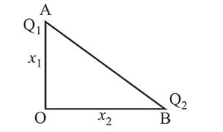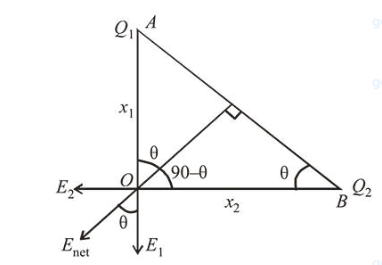# Charges Q1 and Q2 are at points A and B of a right angle triangle OAB (see figure).Question:

Charges $Q_{1}$ and $Q_{2}$ are at points A and B of a right angle triangle OAB (see figure). The resultant electric field at point $\mathrm{O}$ is perpendicular to the hypotenuse, then $Q_{1} / Q_{2}$ is proportional to :1. (1) $\frac{x_{1}{ }^{3}}{x_{2}{ }^{3}}$

2. (2) $\frac{x_{2}}{x_{1}}$

3. (3) $\frac{x_{1}}{x_{2}}$

4. (4) $\frac{x_{2}^{2}}{x_{1}^{2}}$

Correct Option: , 3

Solution:

(3) Electric field due charge $Q_{2}, E_{2}=\frac{k Q_{2}}{x_{2}^{2}}$

Electric field due charge $Q_{1}, E_{1}=\frac{k Q_{1}}{x_{1}^{2}}$From figure,

$\tan \theta=\frac{E_{2}}{E_{1}}=\frac{x_{1}}{x_{2}} \Rightarrow \frac{k Q_{2}}{x_{2}^{2} \times \frac{k Q_{1}}{x_{1}^{2}}}=\frac{x_{1}}{x_{2}}$

$\Rightarrow \frac{Q_{2} x_{1}^{2}}{Q_{1} x_{2}^{2}}=\frac{x_{1}}{x_{2}} \Rightarrow \frac{Q_{2}}{Q_{1}}=\frac{x_{2}}{x_{1}}$ or, $\frac{Q_{1}}{Q_{2}}=\frac{x_{1}}{x_{2}}$